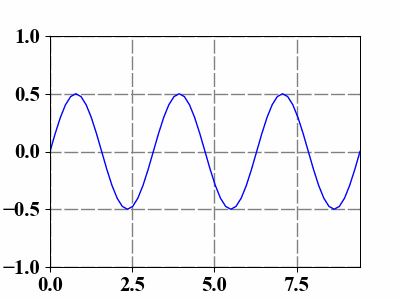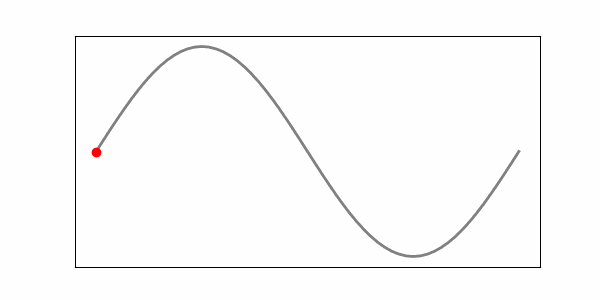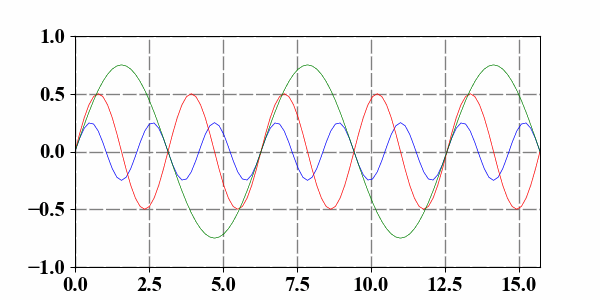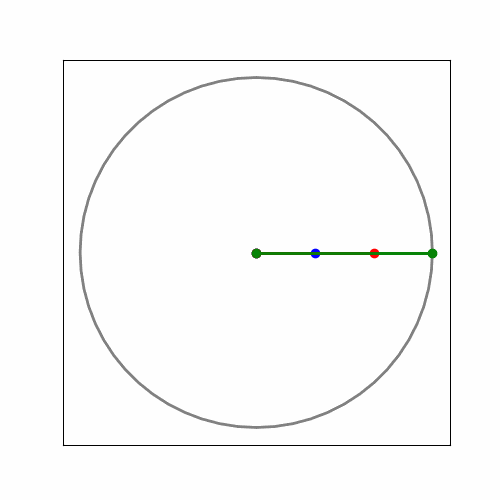### 1 The first animation

Making animations using Python is very convenient and here we first implement the propagation of a sine or cosine function;

  1 2 3 4 5 6 7 8 9 10 11 12 13 14 15 16 17 18 19 20  import numpy as np import matplotlib.pyplot as plt from matplotlib import animation t = np.linspace(0, 3*np.pi, 61) s = np.sin(t) fig = plt.figure(figsize=(6, 3)) line, = plt.plot(t, s, 'b', lw=2) def animate(theta): s = np.sin((t-theta)*2) * 0.5 line.set_data(t, s) return line, ani = animation.FuncAnimation(fig, animate, frames=np.linspace(0, 2*np.pi, 51), interval=100, blit=True) plt.xlim(t, t[-1]); plt.ylim(-1, 1) plt.grid(ls=(2, (10, 2)), lw=1, color='gray') plt.box('off')### 2 How to save the animation

Use writergif = animation.PillowWriter(fps=25); ani.save('Sine.gif', writer=writergif) to save this animation if necessary.

### 3 Data point pursuing

  1 2 3 4 5 6 7 8 9 10 11 12 13 14 15 16 17 18 19 20 21 22 23  import numpy as np import matplotlib.pyplot as plt from matplotlib import animation x0 = 1.5 t = np.linspace(0, 2*np.pi, 61) s = np.sin(t) * 0.5 fig = plt.figure(figsize=(6, 3)) plt.plot(t, s, lw=2, c='gray') line, = plt.plot(0, 0, 'ro') def animate(i): x = t[i] y = s[i] line.set_data(x, y) return line, ani = animation.FuncAnimation(fig, animate, frames=np.arange(len(t)), interval=50, blit=True) plt.xticks([]); plt.yticks([]) plt.box('off')1 2 3 4 5 6 7 8 9 10 11 12 13 14 15 16 17 18 19 20 21 22 23 24 25 26  import numpy as np import matplotlib.pyplot as plt from matplotlib import animation t = np.linspace(0, 5*np.pi, 101) s = np.sin(t) fig = plt.figure(figsize=(6, 3)) ln1, = plt.plot(t, s, 'b', lw=0.5) ln2, = plt.plot(t, s, 'r', lw=0.5) ln3, = plt.plot(t, s, 'g', lw=0.5) def animate(theta): s1 = np.sin((t-2*theta)*3) * 0.25 s2 = np.sin((t-4*theta)*2) * 0.50 s3 = np.sin((t-6*theta)*1) * 0.75 ln1.set_data(t, s1) ln2.set_data(t, s2) ln3.set_data(t, s3) return ln1, ln2, ln3, ani = animation.FuncAnimation(fig, animate, frames=np.linspace(0, 2*np.pi, 51), interval=100, blit=True) plt.xlim(t, t[-1]); plt.ylim(-1, 1) plt.grid(ls=(2, (10, 2)), lw=1, color='gray') plt.box('off')1 2 3 4 5 6 7 8 9 10 11 12 13 14 15 16 17 18 19 20 21 22 23 24 25 26 27 28 29 30 31 32  import numpy as np import matplotlib.pyplot as plt from matplotlib.animation import FuncAnimation x0 = 1.5 t = np.linspace(0, 2*np.pi, 201) x = np.cos(t); y = np.sin(t) fig = plt.figure(figsize=(2, 2)) plt.plot(x, y, lw=3, c='gray') ln1, = plt.plot([], [], 'b-o', lw=2) ln2, = plt.plot([], [], 'r-o', lw=1.5) ln3, = plt.plot([], [], 'g-o', lw=1) ax = plt.gca() ax.spines['top'].set_visible(False) ax.spines['bottom'].set_visible(False) ax.spines['left'].set_visible(False) ax.spines['right'].set_visible(False) def animate(theta): x1 = np.cos(theta) * 0.3; y1 = np.sin(theta) * 0.3 x2 = np.cos(2*theta) * 0.6; y2 = np.sin(2*theta) * 0.6 x3 = np.cos(3*theta); y3 = np.sin(3*theta) ln1.set_data([0, x1], [0, y1]) ln2.set_data([0, x2], [0, y2]) ln3.set_data([0, x3], [0, y3]) return ln1, ln2, ln3, ani = FuncAnimation(fig, animate, frames=np.linspace(0, 2*np.pi, 91), interval=20, blit=True) plt.xticks([]); plt.yticks([])